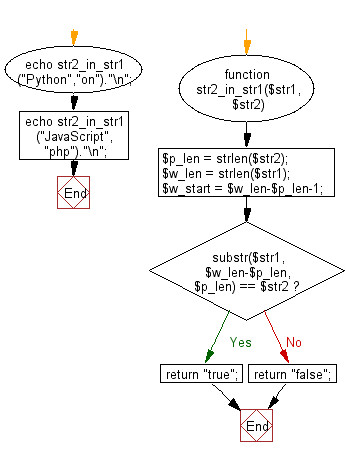﻿ PHP Exercise: Test if a given string occurs at the end of another given string - w3resource# PHP Exercises: Test if a given string occurs at the end of another given string

## PHP: Exercise-36 with Solution

Write a PHP program to test if a given string occurs at the end of another given string.

Sample Solution: -

PHP Code:

``````<?php
function str2_in_str1(\$str1, \$str2) {
\$p_len = strlen(\$str2);
\$w_len = strlen(\$str1);
\$w_start = \$w_len-\$p_len-1;
if (substr(\$str1, \$w_len-\$p_len, \$p_len) == \$str2) {
return "true";
}
else
{
return "false";
}
}
echo str2_in_str1("Python","on")."\n";
echo str2_in_str1("JavaScript","php")."\n";
?>
```
```

Sample Output:

```true
false
```

Flowchart:PHP Code Editor:

Have another way to solve this solution? Contribute your code (and comments) through Disqus.

What is the difficulty level of this exercise?

﻿

## PHP: Tips of the Day

Returning JSON from a PHP Script

While you're usually fine without it, you can and should set the Content-Type header:

Example:

```<?PHP
\$data = /** whatever you're serializing **/;